# 4th Grade Math: The Coordinate Graph Chapter Exam

Exam Instructions:

Choose your answers to the questions and click 'Next' to see the next set of questions. You can skip questions if you would like and come back to them later with the yellow "Go To First Skipped Question" button. When you have completed the practice exam, a green submit button will appear. Click it to see your results. Good luck!

### Page 1

#### Question 1 1. In which quadrant is the point (3, 6)?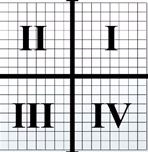#### Question 2 2. In which quadrant is the point (-2, 5)?#### Question 3 3. Use the number line to solve -4 + 6 - 3 + 7.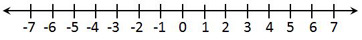### Page 2

#### Question 6 6. The following graph displays which point?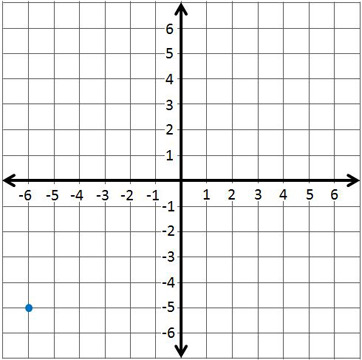#### Question 8 8. What are the coordinates of this point?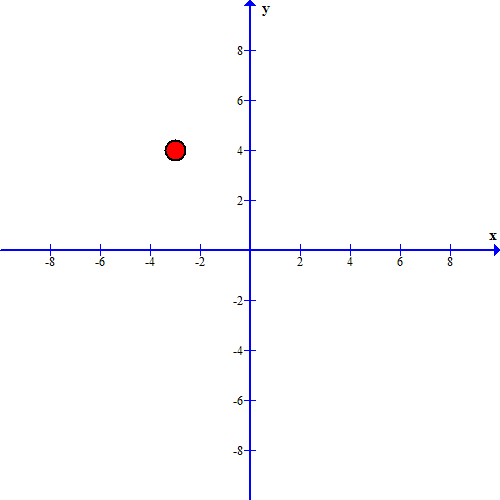#### Question 9 9. The following graph displays which point?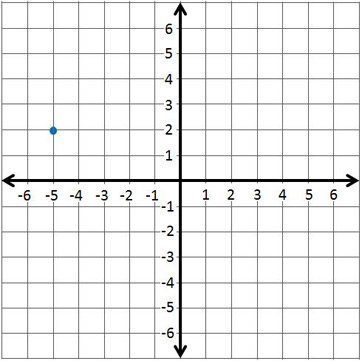### Page 3

#### Question 13 13. Use the number line to solve 9 - 13 + 7 - 3.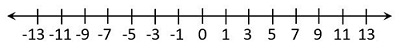#### Question 14 14. The following graph displays which point?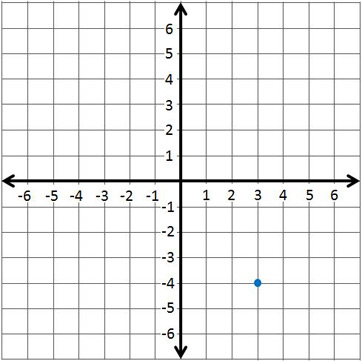### Page 4

#### Question 17 17. Use the number line to solve -5 - 2 + 3.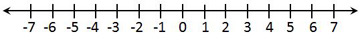#### Question 18 18. What number does the point on this line represent?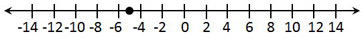#### 4th Grade Math: The Coordinate Graph Chapter Exam Instructions

Choose your answers to the questions and click 'Next' to see the next set of questions. You can skip questions if you would like and come back to them later with the yellow "Go To First Skipped Question" button. When you have completed the practice exam, a green submit button will appear. Click it to see your results. Good luck!

Support